# How to Use Excel ROUND, ROUNDUP, ROUNDDOWN, MROUND & CEILING Functions

Excel provides multiple functions for rounding numbers in one’s worksheet. The most often utilized are namely the ROUND Function, the ROUNDUP Function, the ROUNDDOWN Function, the MROUND Function and the CEILING Function.

The ROUND Function is used to round a number to a certain number of digits/decimal places, specified by the user. The syntax of the ROUND Function is: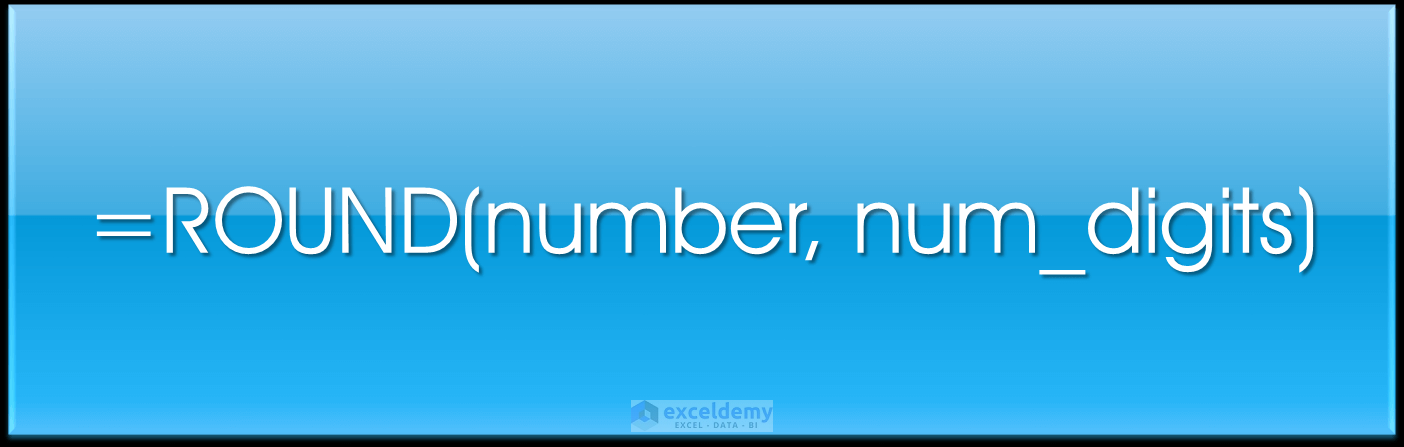where number is the number at hand that you would like to round, and num_digits is the number of digits one wants to round the number by. Both arguments used by the function are required. For any num_digits above 0, the number is then rounded to the decimal places specified by the number of digits. If num_digits is 0 exactly, then the number is rounded to the nearest integer/whole number. In the case where num_digits is less than 0, then the actual number is rounded to the left of the decimal point.

The ROUNDUP Function is used in cases where you want to round a number up, away from 0. The syntax of the ROUNDUP Function is: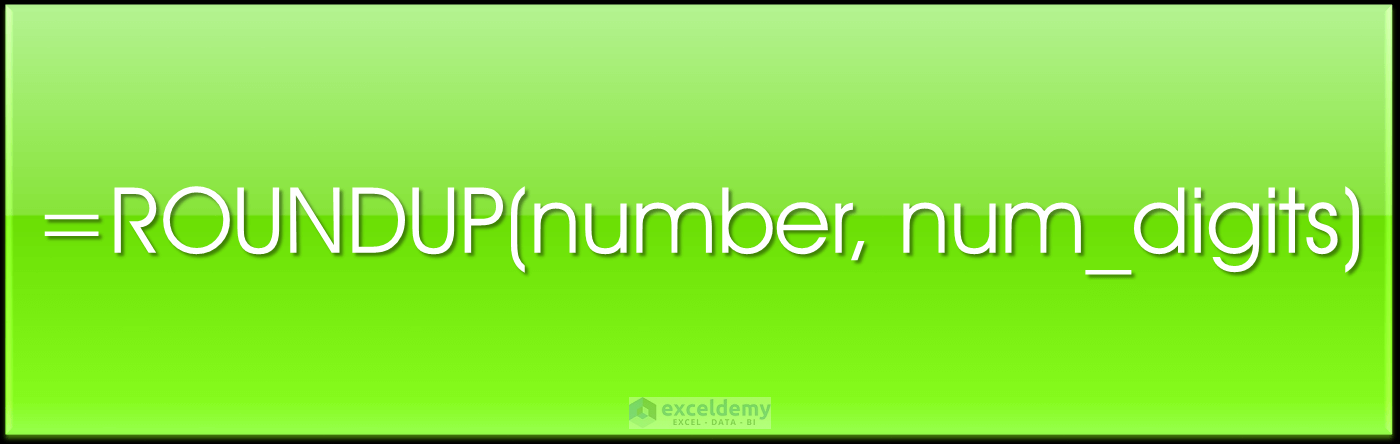where the number is the number at hand that you would like to round up, and the num_digits is the number of digits that you would like to round the number at hand, by. Both arguments used are required for this function. In the case where num_digits is above 0, the number is always rounded up to the decimal places specified. If num_digits is 0 exactly then the number is rounded up to the nearest integer/whole number. If num_digits is less than 0, then the number is rounded up to the left of the decimal point.

The ROUNDDOWN Function, on the other hand, is used to round a number down towards 0. The syntax of the ROUNDDOWN Function is: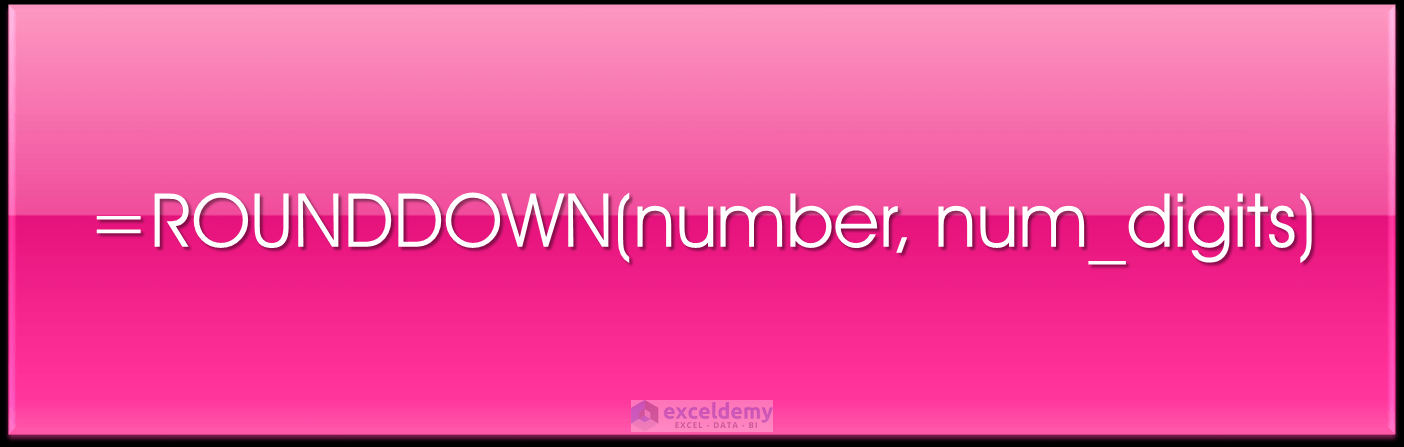where the number is the number that you would like to round down, and the num_digits is the number of digits that you would like to round the number at hand by. Both arguments are required for this function. In this case, if the num_digits value is above 0, then the number value is rounded down to the specified number of decimal places. If num_digits is exactly 0, then the number value is rounded down to the nearest integer/whole number. If num_digits is less than 0, then the number value is rounded down to the left of the decimal point.

The MROUND Function is used to round a number to a desired multiple. The syntax of the MROUND Function is: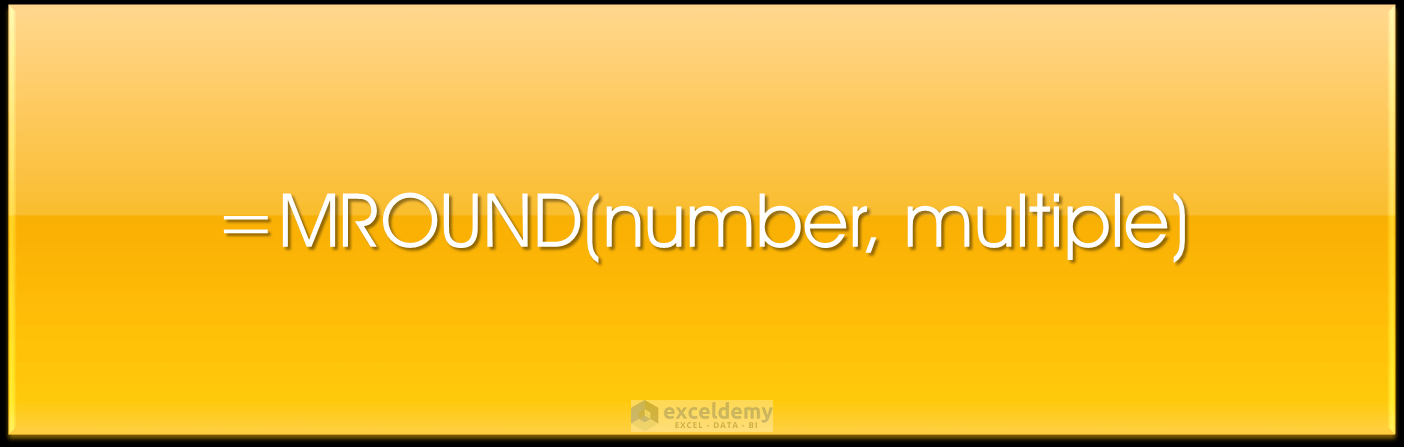where the number is the value that you would like to round, and ‘multiple’ refers to the multiple to which you want to round the number. Both arguments are required.

The CEILING Function is used to round a number up away from zero to the nearest multiple of significance specified. The syntax of the CEILING Function is: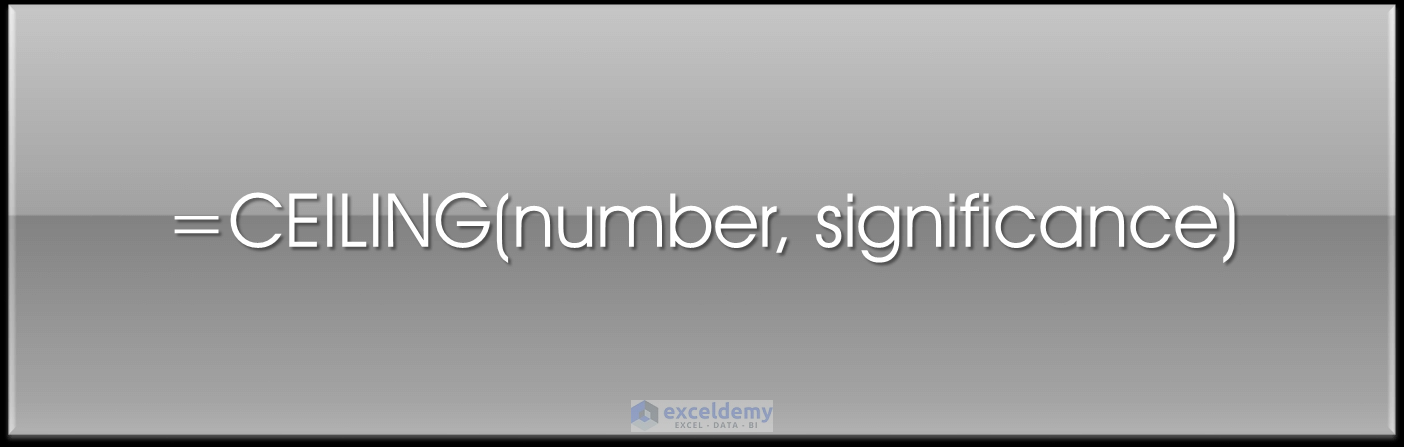where the number is the number that you want to round and significance specifies the multiple to which you want to round up to.

When the number value and the multiple of significance given are the same, no rounding occurs or if the number is a multiple of the multiple of significance. If both the number specified is negative and the significance is negative then the number value is rounded down away from 0. If the number is negative and the significance is positive, then the value is rounded up towards zero.

So, let’s get started with a few simple examples to illustrate the functionality of the rounding functions.

## Using the ROUND Function

We have a list of numbers shown in our source data, that we would like to round to one decimal place, and then two decimal places using the ROUND Function. The source data is shown below.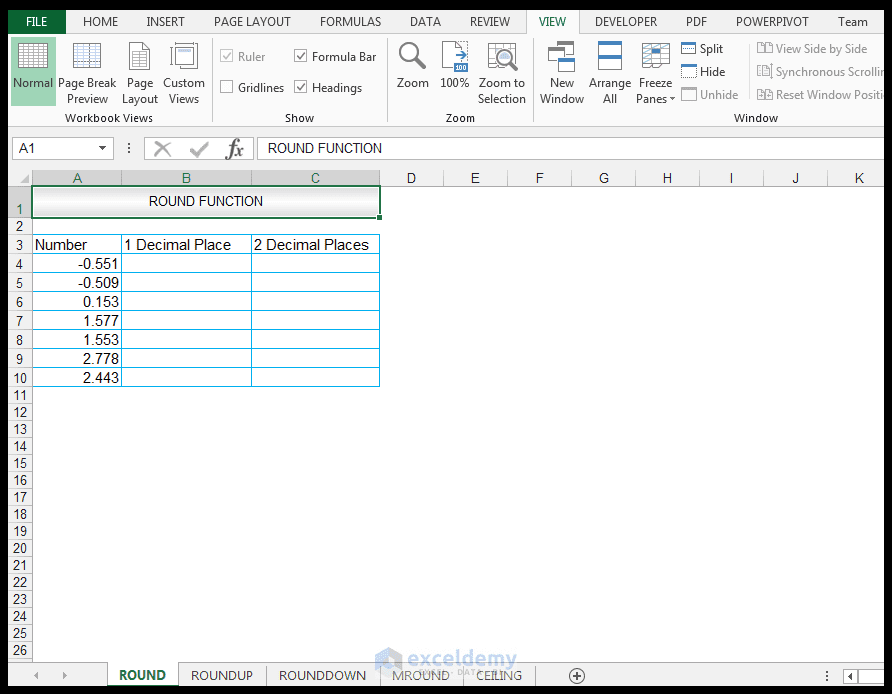1) For one decimal place, in cell B4, we enter the following formula:

=ROUND(A4,1)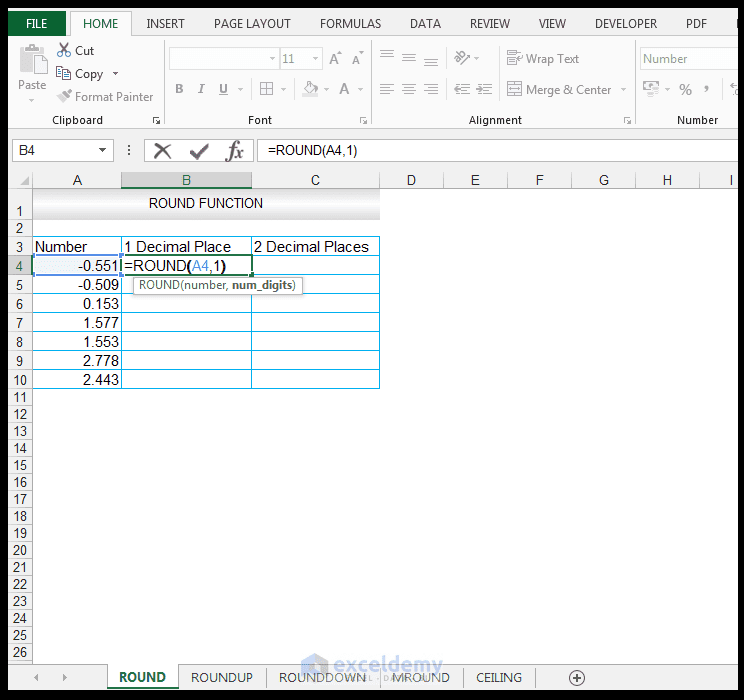2) Upon pressing CTRL-ENTER, we get a value of -0.6 returned.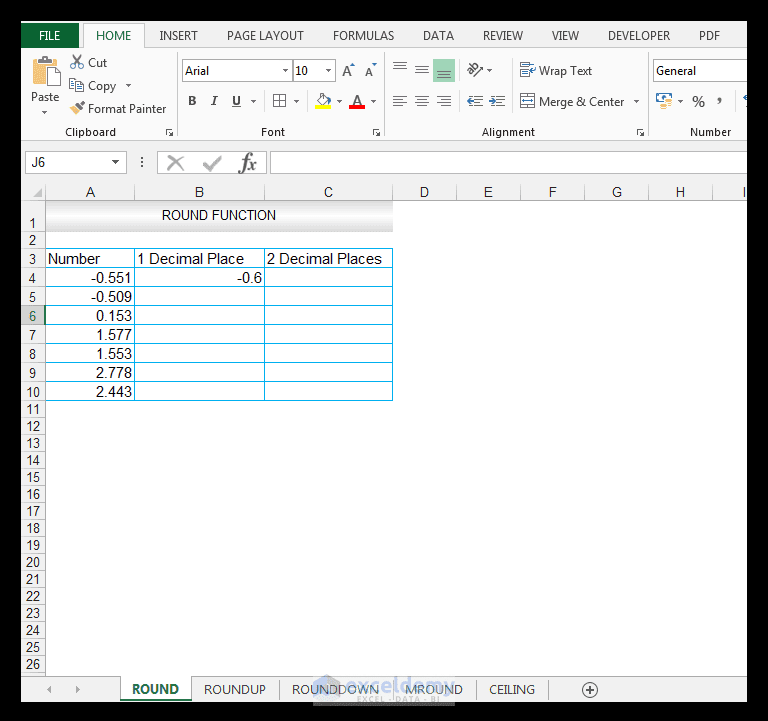3) The value is rounded to one decimal place, specification.

4) Dragging the formula down results in the following results for the rest of the input numbers.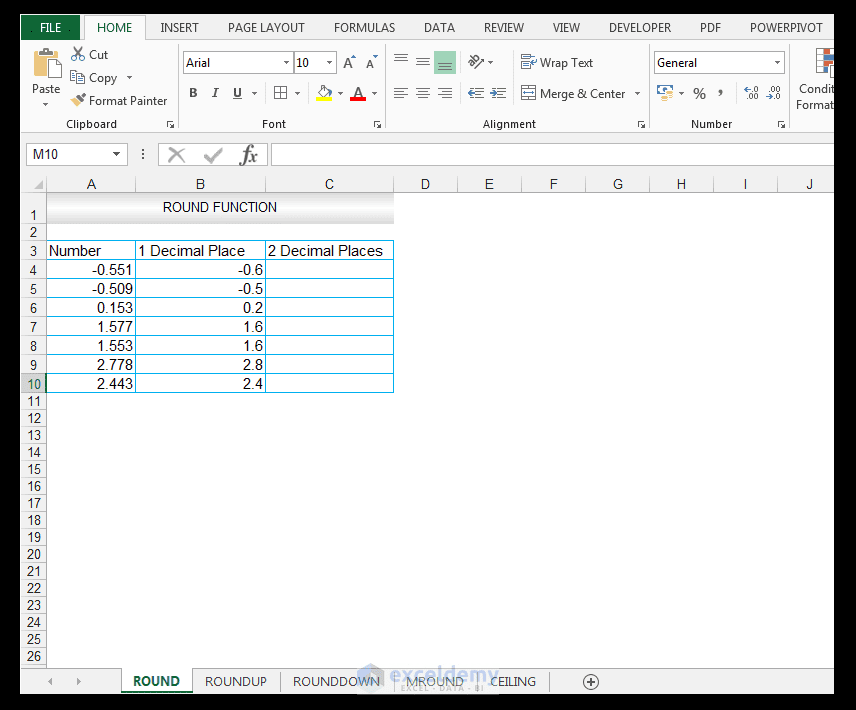5) Note the results the ROUND Function produces for one decimal place for 2.778 which is rounded to 2.8 and 2.443 which is rounded to 2.4.

6) We now want to evaluate what the effect of rounding to two decimal places using the ROUND Function will be, so in cell C4, we enter the following formula.

=ROUND(A4,2)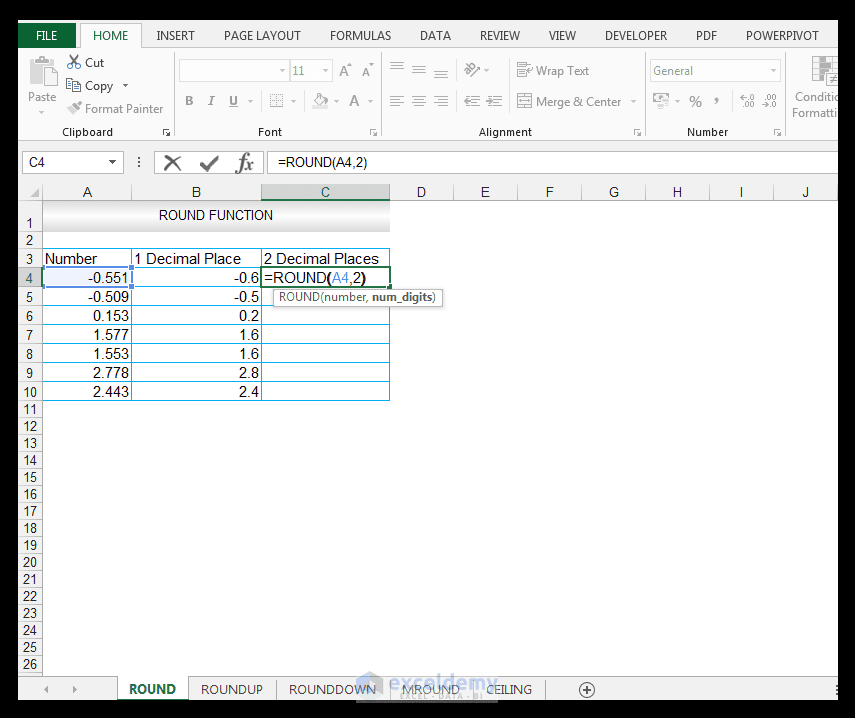7) Upon pressing CTRL-ENTER we get a value of -0.55 returned.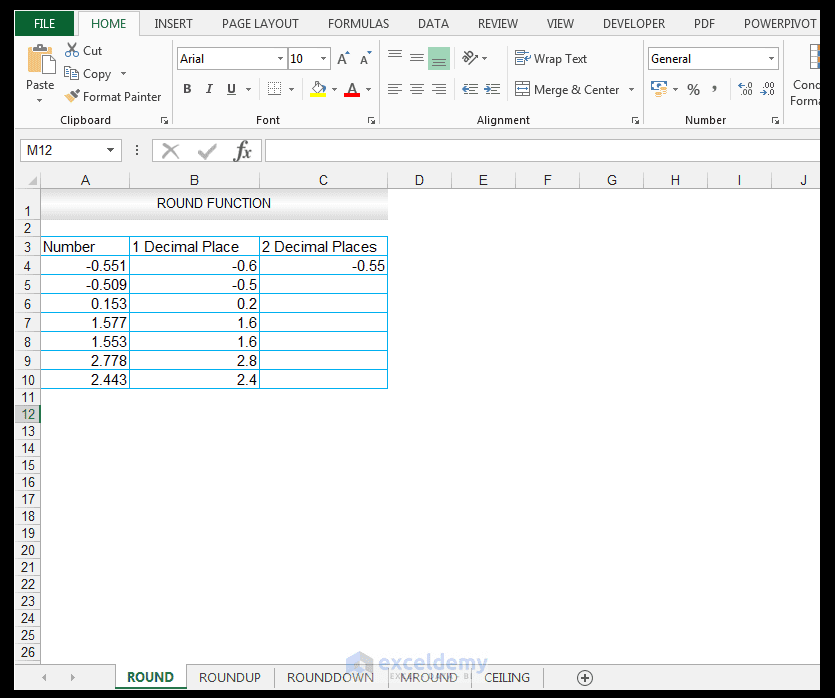8) The value is now rounded to two decimal places specification.

9) Dragging the formula results in the following results for the rest of the input numbers.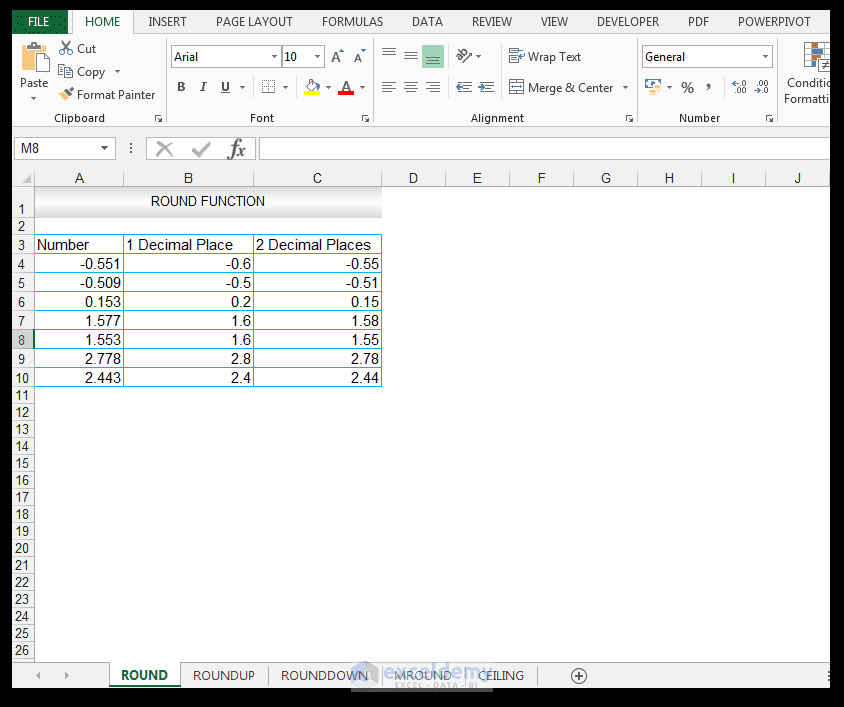10) Note the results the ROUND Function produces for two decimal places for 2.778 is 2.78 and for 2.443 is 2.44.

The numbers 1, 2,3,4 get rounded down when using the ROUND function, as shown for the two decimal place rounding of 2.443 to 2.44.  The numbers 5,6,7,8 get rounded up, as demonstrated for the two decimal place rounding of 2.778 to 2.78.

## Using the ROUNDUP Function

We have a list of numbers shown in our source data, that we would like to round up to one decimal place, two decimal places and 0 number of digits using the ROUNDUP Function. The source data is shown below.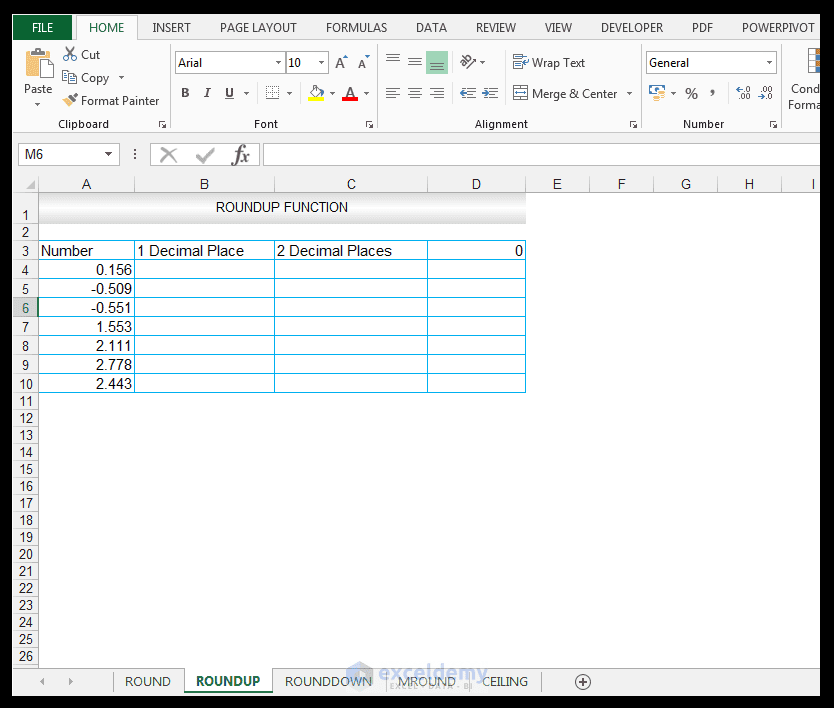1) For one decimal place, in cell B4, enter the following formula:

=ROUNDUP(A4,1)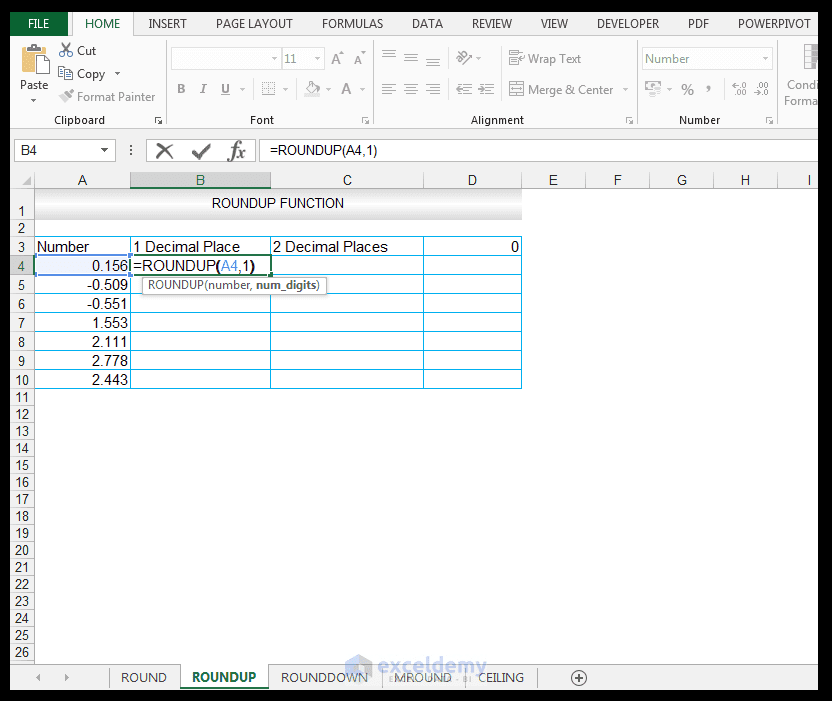2) Upon pressing CTRL-ENTER a value of 0.2 is returned.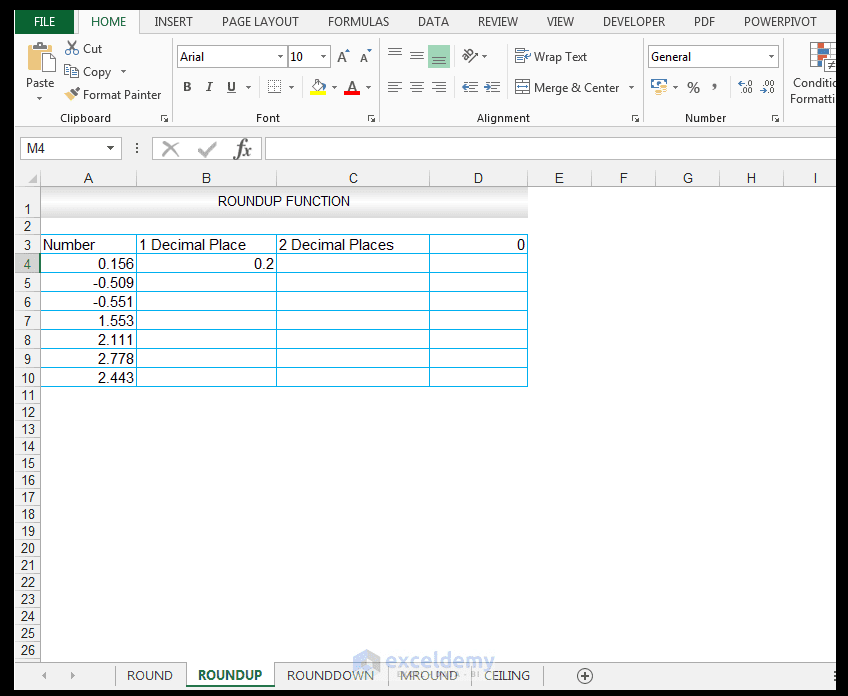3) The value is now rounded up, to one decimal place specification.

4) Dragging the formula results in the following results for the rest of the input numbers.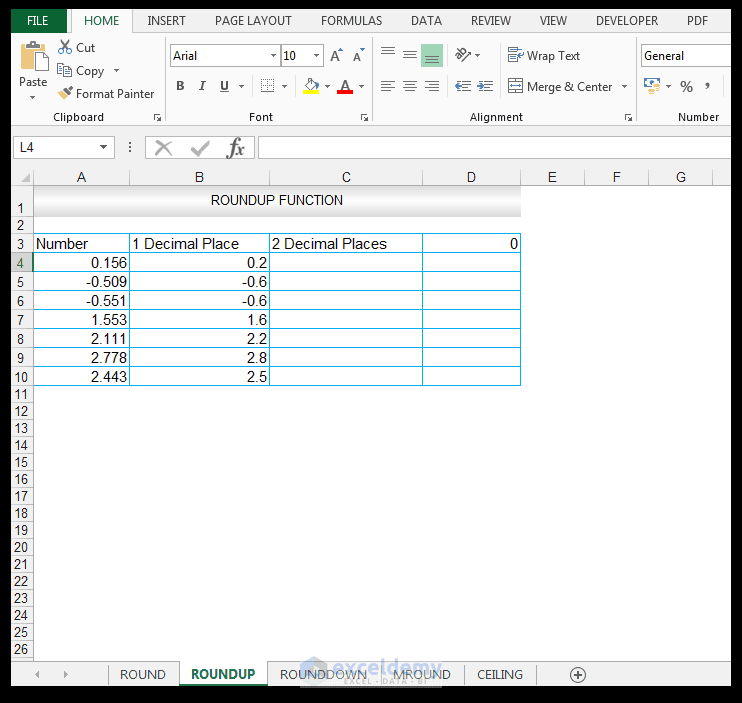5) Note the results the ROUNDUP Function produces for one decimal place for 2.443. The value 2.443 is rounded up to 2.5 using the ROUNDUP Function whereas using the ROUND Function, rounding 2.443 to one decimal place yielded 2.4.

6) We now want to evaluate what the effect of rounding to two decimal places using the ROUNDUP Function will be, so in cell C4, we enter the following formula:

=ROUNDUP(A4,2)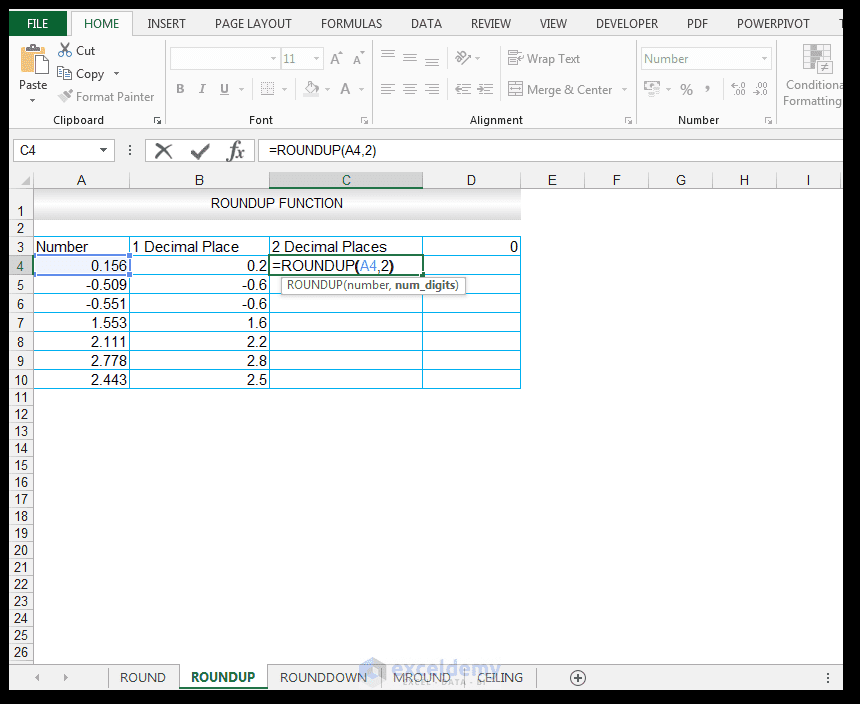7) Upon pressing CTRL-ENTER, we get a value of 0.16 returned.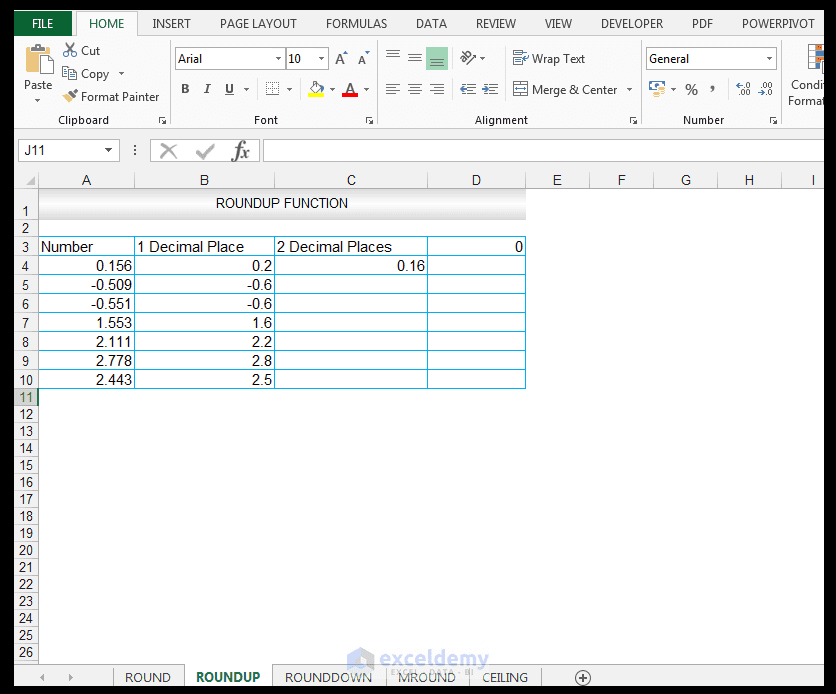8) The value is now rounded up to two decimal places, specification.

9) Dragging the formula results in the following results for the rest of the input numbers.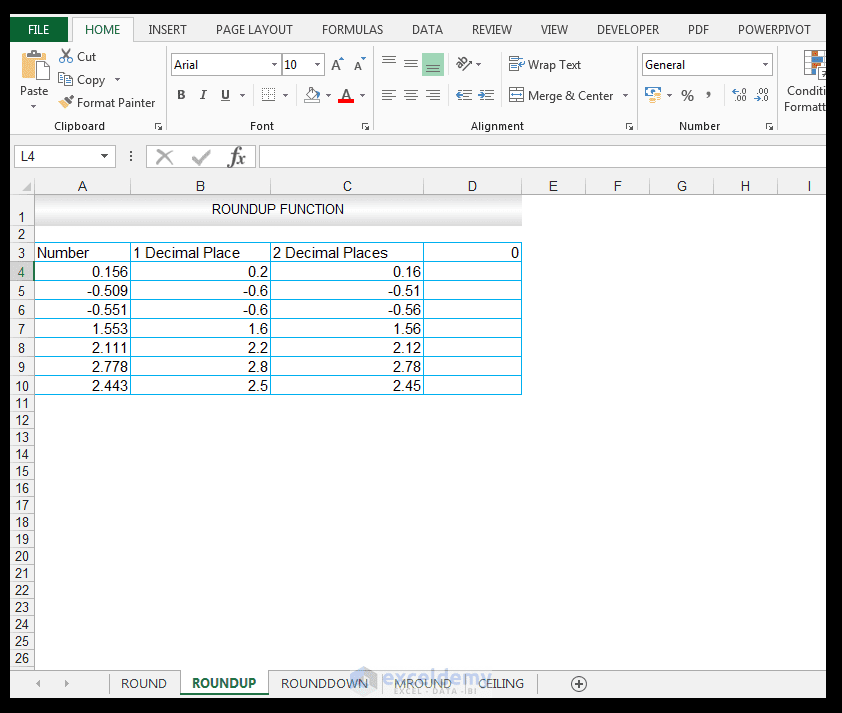10) Note the results the ROUNDUP Function produces for two decimal places for 2.443.The contrast in using the ROUNDUP Function for 2.443 is also seen, for two decimal places, since using the ROUNDUP Function for 2.443 produces 2.45 whereas using the ROUND Function for two decimal places for 2.443 produces 2.44.

The ROUNDUP Function by default rounds up.

11) For no decimal places, or 0 number of digits, in cell D4, we enter the following formula:

=ROUNDUP(A4,0)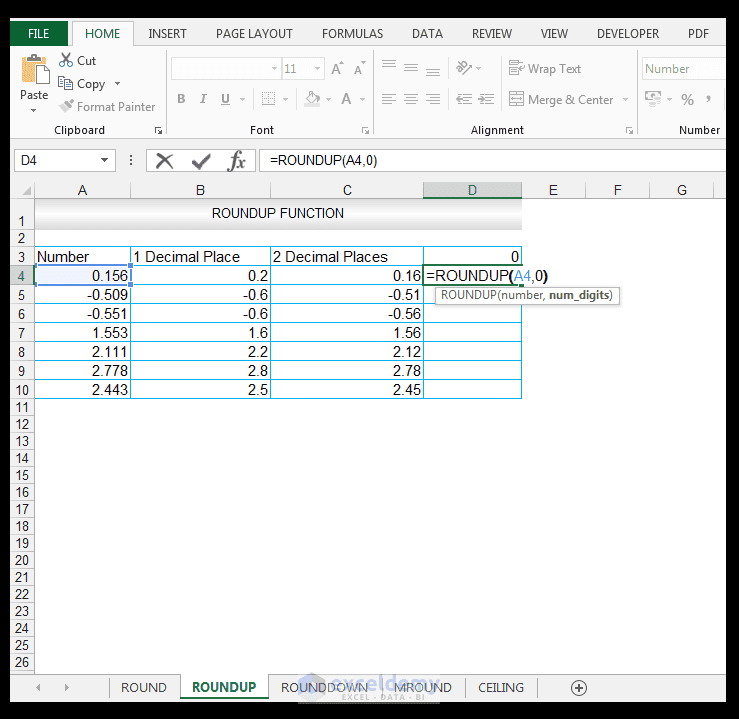12) Upon pressing CTRL-ENTER, the value of 1 is returned as shown below.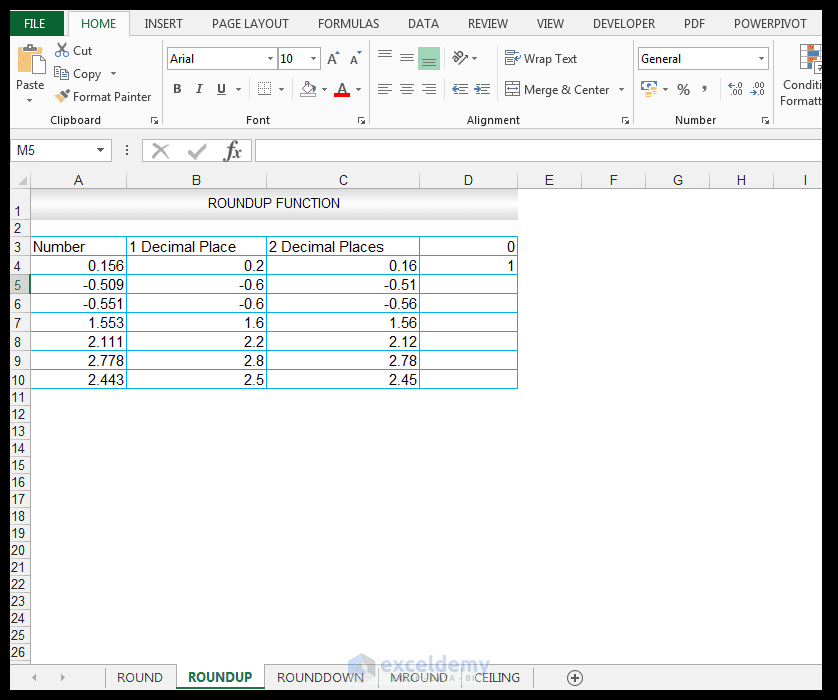13) The value is now rounded up to the nearest integer/whole number which is 1, in this case.

14) Dragging the formula results in the following results for the rest of the input numbers.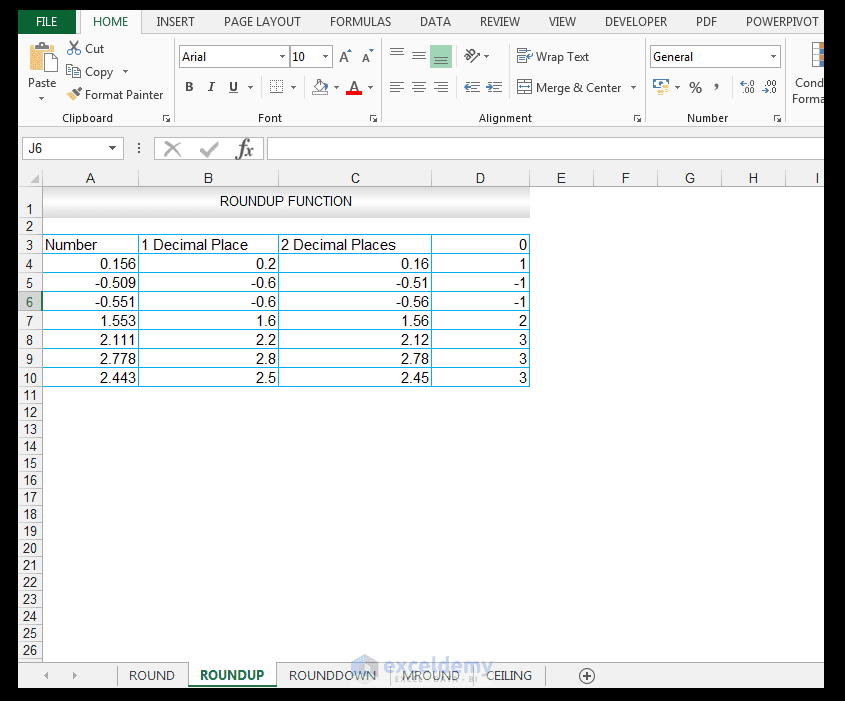15)  Note the results the ROUNDUP Function produces for no decimal places.

## Using the ROUNDDOWN Function

We have a list of numbers shown in our source data, that we would like to round down to one decimal place, two decimal places and 0 number of digits using the ROUNDDOWN Function. The source data is shown below, which is the same source as the ROUNDUP Function.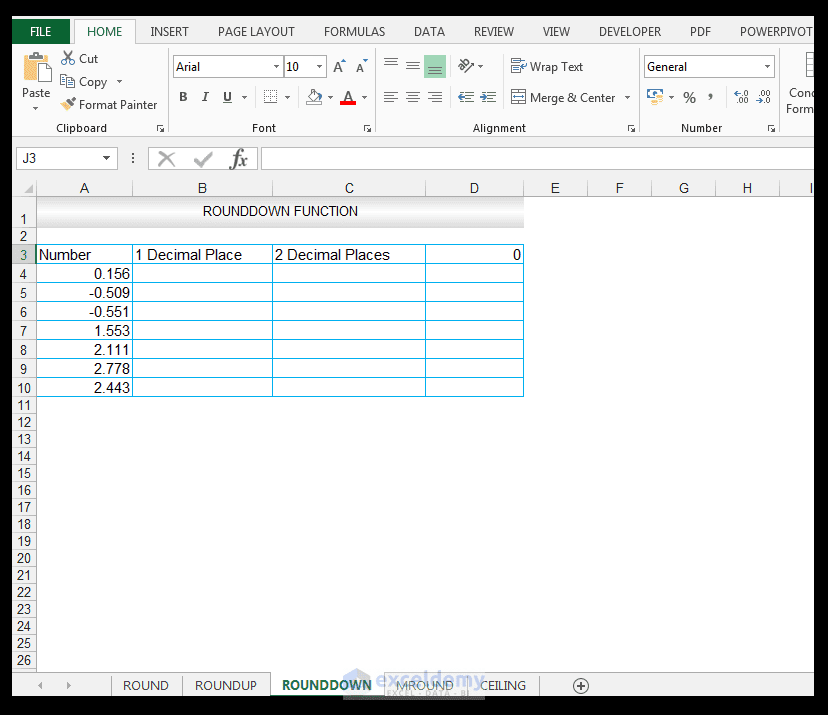1) For one decimal place, in cell B4, enter the following formula.

=ROUNDDOWN(A4,1)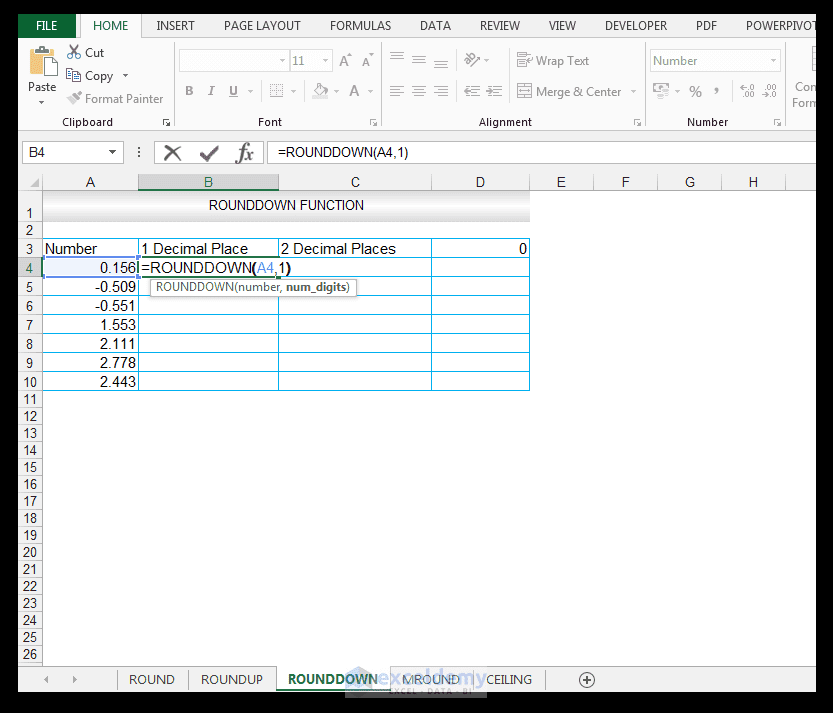2) Upon pressing CTRL-ENTER a value of 0.1 is returned.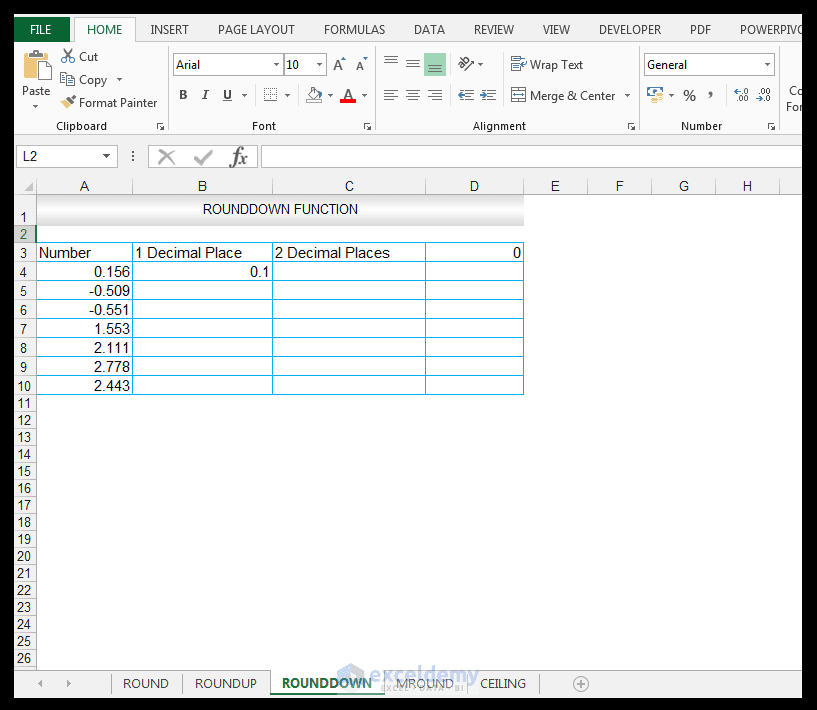3) The value is now rounded down to one decimal place specification.

4) Dragging the formula results in the following results for the rest of the input numbers.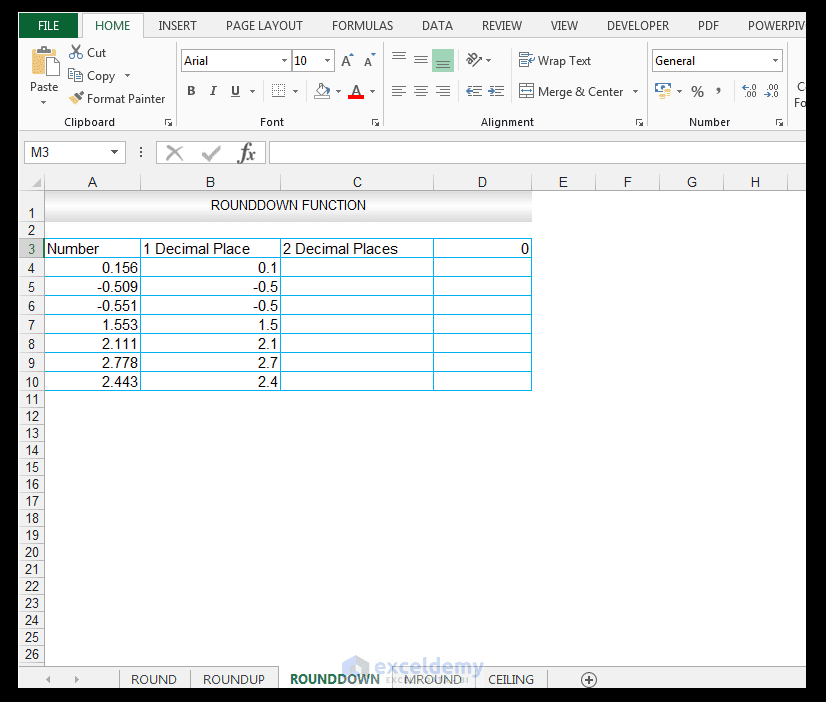5) Note the results the ROUNDDOWN Function produces for one decimal place for 2.443, which is 2.4. Whereas, the value 2.443 is rounded up to 2.5 using the ROUNDUP Function whereas using the ROUND Function, rounding 2.443 to one decimal place yielded 2.4. Note also the results the ROUNDDOWN Function produces for one decimal place for 2.778, which is rounded down to 2.7, whereas the value 2.778 is rounded up to 2.8 by the ROUNDUP Function and 2.8 by the ROUND Function.

6) We now want to evaluate what the effect of rounding to two decimal places using the ROUNDDOWN Function will be, so in cell C4, we enter the following formula.

=ROUNDDOWN(A4,2)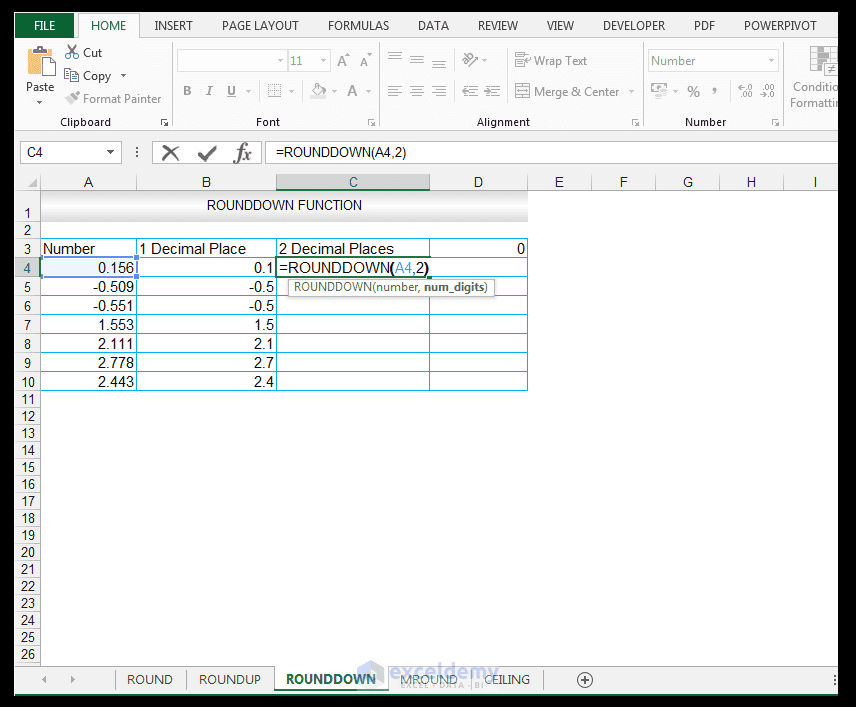7) Upon pressing CTRL-ENTER a value of 0.15 is returned.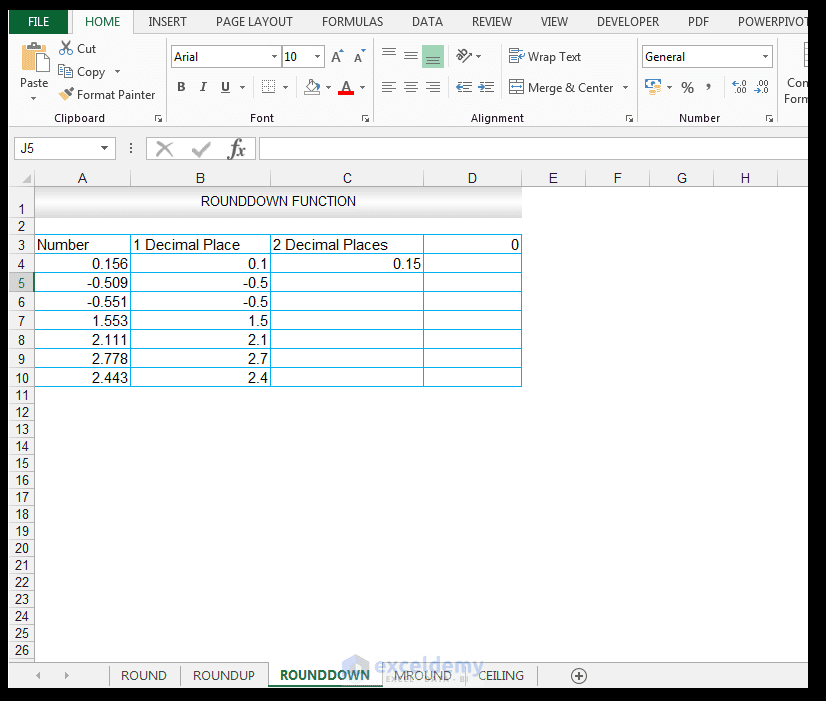8) The value is now rounded down, to two decimals places specification.

9) Dragging the formula results in the following results for the rest of the input numbers.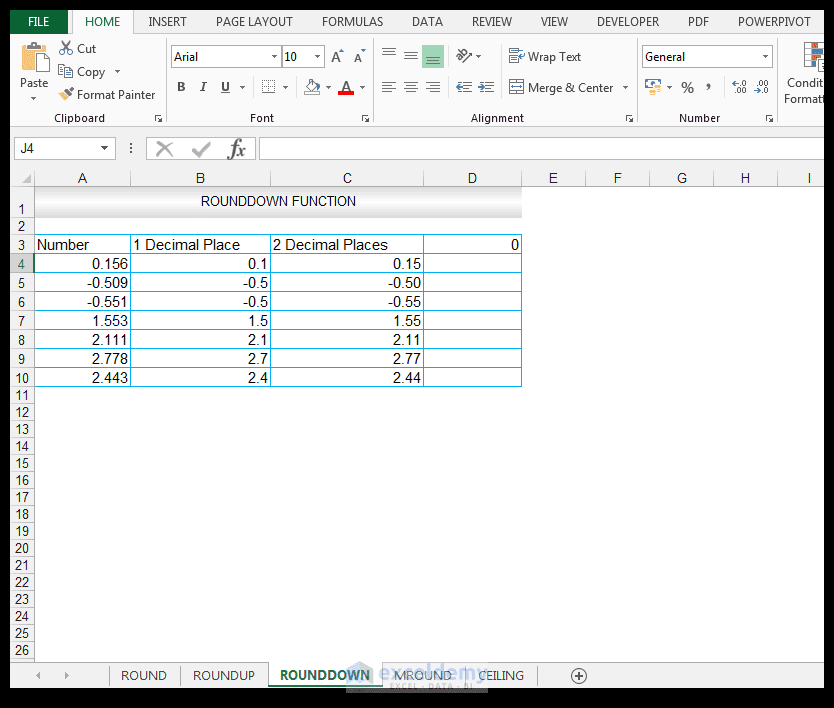10) Note the results the ROUNDDOWN Function produces for two decimal places for 2.443, which is 2.44.The contrast in using the ROUNDUP Function for 2.443 is also seen, for two decimal places, since using the ROUNDUP Function for 2.443 produces 2.45 whereas using the ROUND Function for two decimal places for 2.443 produces 2.44.

The ROUNDDOWN Function by default rounds down

11) For no decimal places, or 0 value or 0 number of digits, in cell D4, we enter the following formula:

=ROUNDDOWN(A4,0)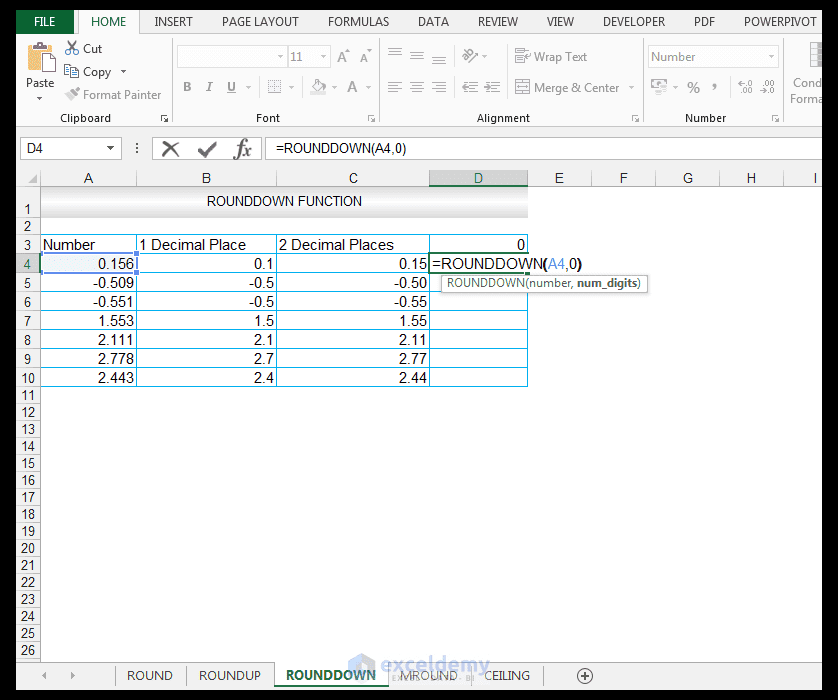12) Upon pressing CTRL-ENTER, the value of 0 is returned as shown below.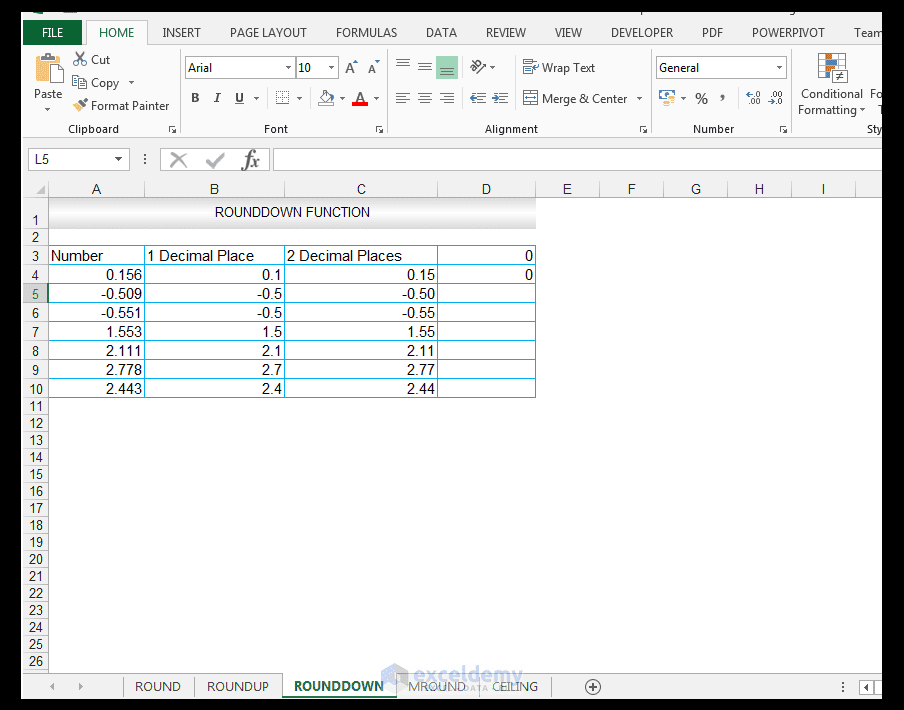13) The value is now rounded down to the nearest integer/whole number which is 0, in this case.

14) Dragging the formula results in the following results for the rest of the input numbers.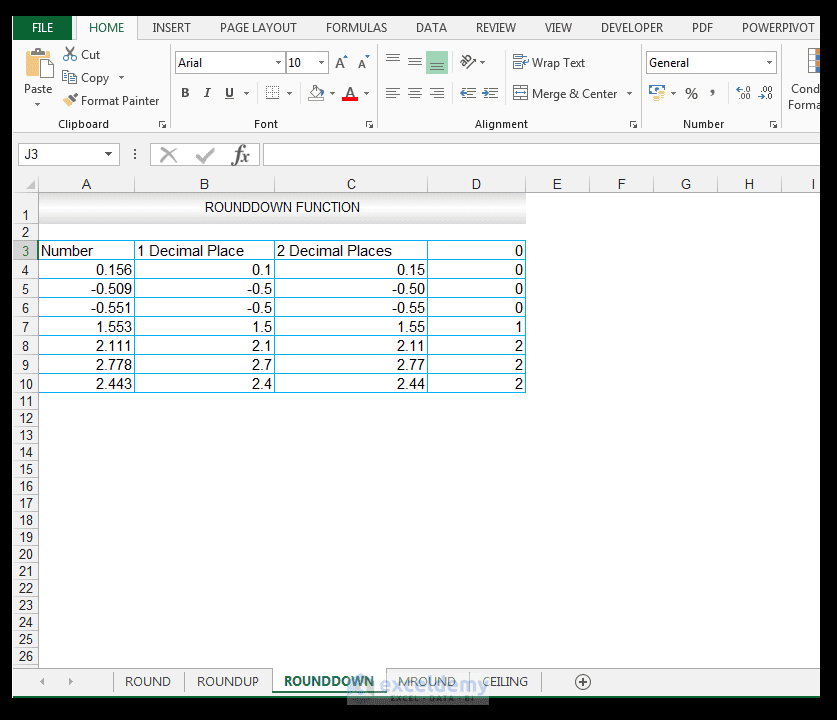15)  Note the results the ROUNDDOWN Function produces for no decimal places.

## Using the MROUND Function

We have a list of numbers shown in our source data in column A, that we would like to round to the nearest multiple specified in column B. The source data is shown below.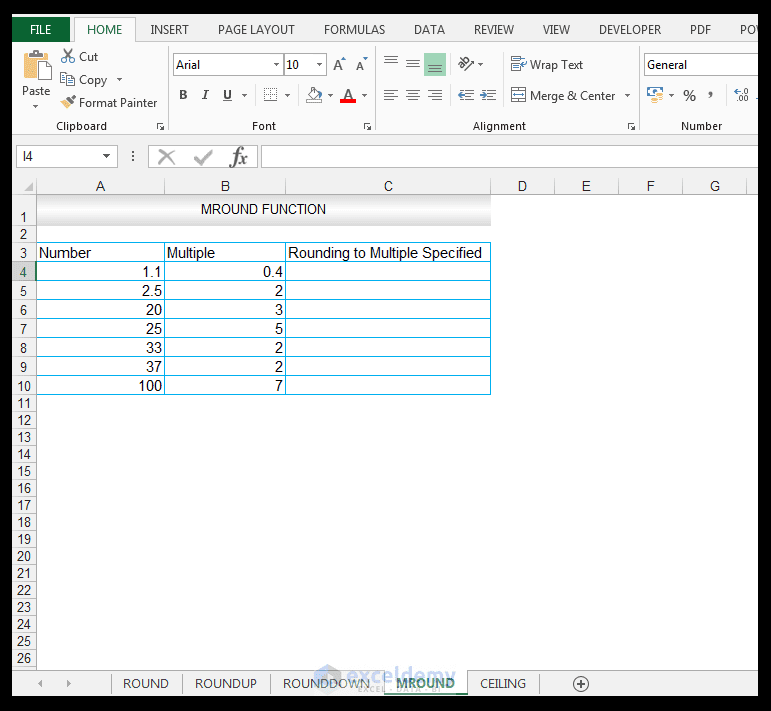1) So, in cell C4 enter the following formula.
=MROUND(A4,B4)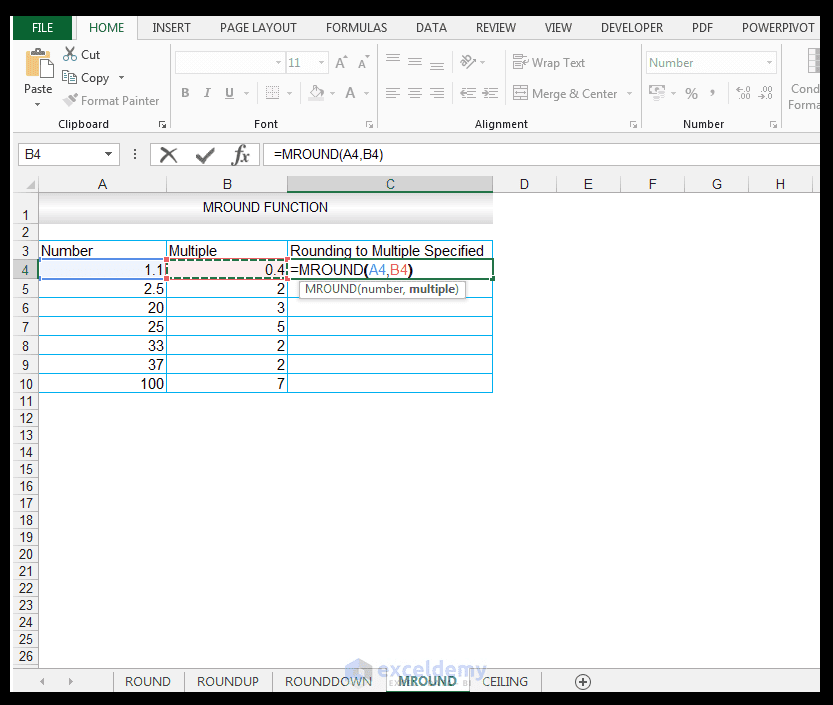2) Upon pressing CTRL-ENTER a value of 1.2 is returned, which is the closest multiple of 0.4 going from the value of 1.1. In this case, the MROUND Function rounded up.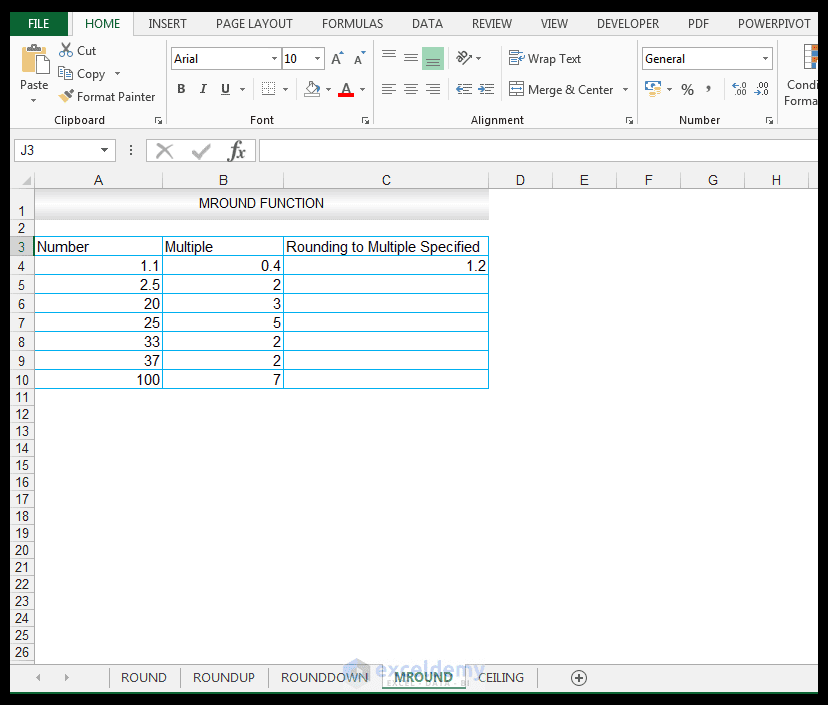3) Dragging the formula results in the following results for the rest of the input numbers.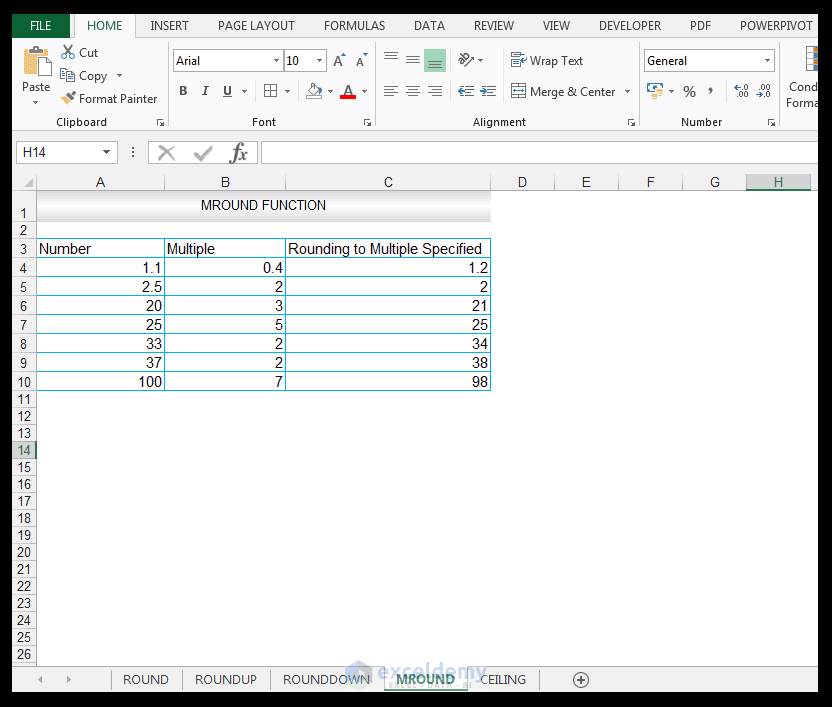Note in the case of 2.5, the nearest multiple of 2, would be 2 (0.5 away) since the next multiple of 2 is only 4 which is 1.5 away from 2.5. In this case the MROUND Function rounded down.

### Using the CEILING Function

We have a list of numbers shown in our source data in column A, that we would like to round up to the nearest multiple of significance specified in column B. The source data is shown below and is the same data used for the MROUND FUNCTION.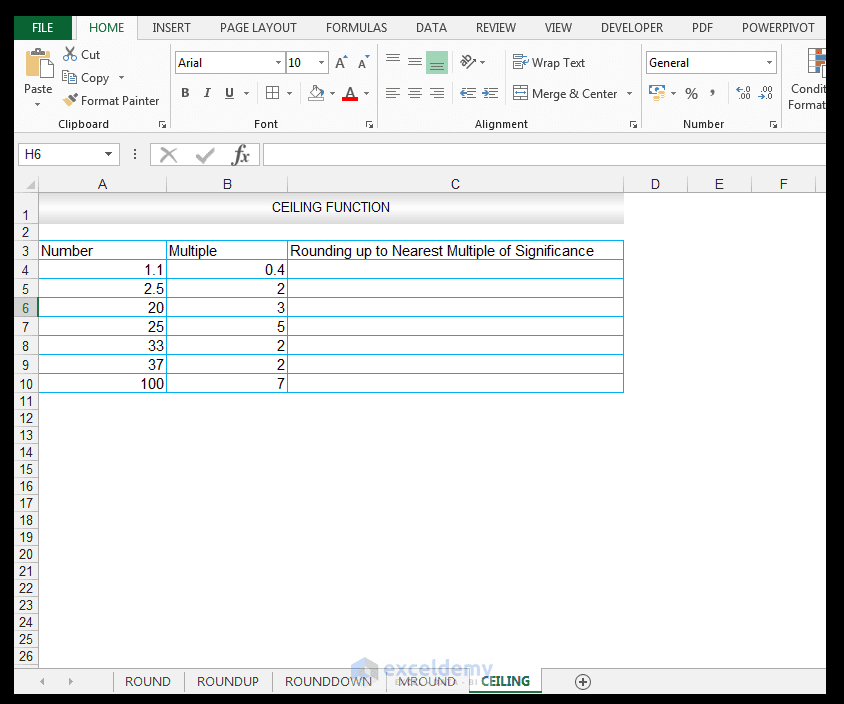1) So, in cell C4 enter the following formula.

=CEILING(A4,B4)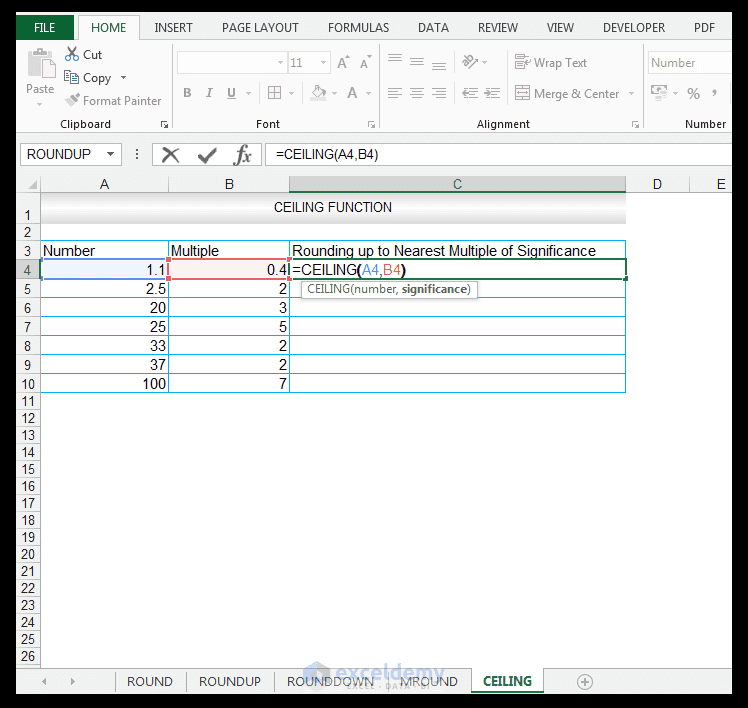2) Upon pressing CTRL-ENTER a value of 1.2 is returned, which is the value rounded up to the nearest multiple of significance.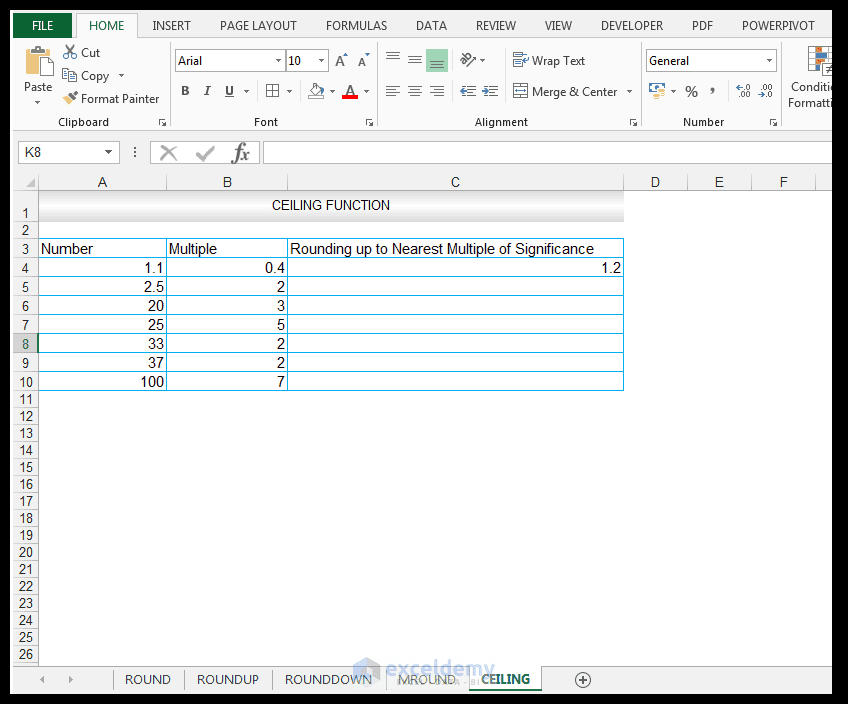3) Dragging the formula results in the following results for the rest of the input numbers.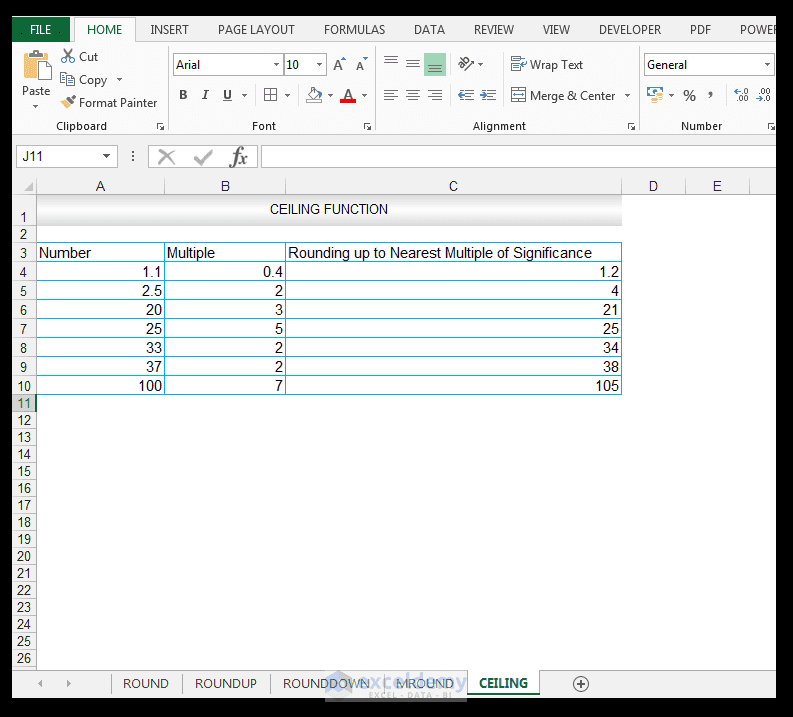Note in the case of 2.5, the value is rounded up to the nearest multiple of 2, which is 4. This is different to the value returned using the MROUND Function, which returned a value of 2 for 2.5, using 2 as a multiple.

And there you have it.

HowToUseRoundRoundUpRoundDownMRoundAndCeilingFunction

## Conclusion

Excel provides different functions to round numbers, you can select a suitable function depending on your needs, requirements or industry dependent specifications. One must remember though that the rounding functions change the actual number value, so when utilized in formulas, it’s the rounded number that is input, and not the original source number.